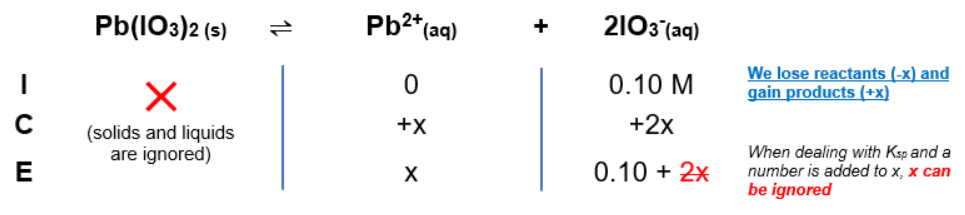# Problem: The solubility of Pb(IO3)2(s) in a 0.10-M KIO3 solution is 2.6 X 10 -11 mol/L. Calculate Ksp for Pb(IO3)2(s).

###### FREE Expert Solution

ICE Table:Ksp expression:

$\overline{){{\mathbf{K}}}_{{\mathbf{sp}}}{\mathbf{=}}\frac{\mathbf{products}}{\overline{)\mathbf{reactants}}}{\mathbf{=}}{\mathbf{\left[}}{{\mathbf{Pb}}}^{\mathbf{2}\mathbf{+}}{\mathbf{\right]}}{\mathbf{\left[}}{{{\mathbf{IO}}}_{{\mathbf{3}}}}^{{\mathbf{-}}}{{\mathbf{\right]}}}^{{\mathbf{2}}}}$

100% (239 ratings)###### Problem Details

The solubility of Pb(IO3)2(s) in a 0.10-M KIO3 solution is 2.6 X 10 -11 mol/L. Calculate Ksp for Pb(IO3)2(s).

Frequently Asked Questions

What scientific concept do you need to know in order to solve this problem?

Our tutors have indicated that to solve this problem you will need to apply the Ksp concept. You can view video lessons to learn Ksp. Or if you need more Ksp practice, you can also practice Ksp practice problems.

What professor is this problem relevant for?

Based on our data, we think this problem is relevant for Professor Dumont's class at McMaster University.

What textbook is this problem found in?

Our data indicates that this problem or a close variation was asked in Chemistry: An Atoms First Approach - Zumdahl Atoms 1st 2nd Edition. You can also practice Chemistry: An Atoms First Approach - Zumdahl Atoms 1st 2nd Edition practice problems.